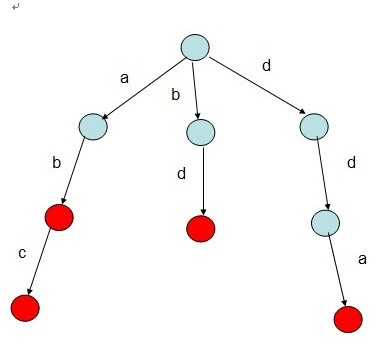# 【模板】Trie树模板Trie树，是一种树形结构，是一种哈希树的变种。典型应用是用于统计，排序和保存大量的字符（但不仅限于字符串），所以经常被搜索引擎系统用于文本词频统计。

int num = 26;  //以26个小写字母为例
struct TrieNode
{
bool isTri;
TrieNode *next[num];
};

void Insert(TrieNode* root,const char* word)
{
TrieNode *now = root;
while(*word)
{
if(now->next[*word-'a']==NULL)              //*word-'a'用于表示某一字符的位置
{
TrieNode *newNode = new TrieNode();
newNode->isTri = false;
newNode->yes = 0;
memset(newNode->next,NULL,sizeof(newNode->next));

now->next[*word-'a'] = newNode;
}
now = now->next[*word-'a'];
word++;
}
now->isTri = true;
}

bool Search(TrieNode* root,const char* word)
{
TrieNode *now = root;
while(*word)
{
if(*word&&now != NULL)
{
now = now->next[word-'a'];
word++;
}
}
return (now->isTri&&now!=NULL);
}

void Delete(TrieNode* node)
{
for(int i = 0;i<num;i++)
{
if(node->next[i]!=NULL)
{
Delete(node->next[i]);
}
}
delete node;
}

#include<cstdio>
#include<cstring>
#include<iostream>
#include<algorithm>
using namespace std;
const int num = 26;

struct TrieNode
{
bool isTri;
TrieNode *next[num];
}

void Insert(TrieNode* root,const char* word)
{
TrieNode *now = root;
while(*word)
{
if(now->next[word-'a']==NULL)
{
TrieNode *newNode = new TrieNode();
newNode->isTri = false;
memset(newNode->next,NULL,memset(newNode->next));

now->next[word-'0'] = newNode;
}
now = *newNode;
word++;
}
now->isTri = true;
}

bool Search(TrieNode* root,const char* word)
{
TrieNode *now = root;
while(*word)
{
if(*word&&now != NULL)
{
now = now->next[word-'a'];
word++;
}
}
return (now->isTri&&now!=NULL);
}

void Delete(TrieNode* node)
{
for(int i = 0;i<num;i++)
{
if(node->next[i]!=NULL)
{
Delete(node->next[i]);
}
}
delete node;
}
int main()
{
TrieNode *root = new TrieNode();
root->isTri = false;
memset(root->next,NULL,sizeof(root->next));
//主函数就不写了，这里就是应用数据结构的地方,交代一下根节点的初始化即可
return 0;
}